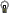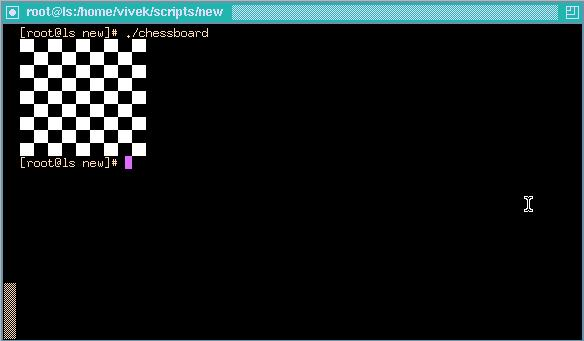Linux Shell Scripting Tutorial (LSST) v1.05r3 Prev Chapter 3: Shells (bash) structured Language Constructs Next

# for Loop

Syntax:
```            for { variable name } in { list }
do
execute one for each item in the list until the list is
not finished (And repeat all statement between do and done)
done
```

Before try to understand above syntax try the following script:

 `\$ cat > testfor for i in 1 2 3 4 5 do echo "Welcome \$i times" done`

Run it above script as follows:
\$ chmod +x testfor
\$ ./testfor

The for loop first creates i variable and assigned a number to i from the list of number from 1 to 5, The shell execute echo statement for each assignment of i. (This is usually know as iteration) This process will continue until all the items in the list were not finished, because of this it will repeat 5 echo statements. To make you idea more clear try following script:

 `\$ cat > mtable #!/bin/sh # #Script to test for loop # # if [ \$# -eq 0 ] then echo "Error - Number missing form command line argument" echo "Syntax : \$0 number" echo "Use to print multiplication table for given number" exit 1 fi n=\$1 for i in 1 2 3 4 5 6 7 8 9 10 do echo "\$n * \$i = `expr \$i \* \$n`" done`

Save above script and run it as:
\$ chmod 755 mtable
\$ ./mtable 7
\$ ./mtable
For first run, above script print multiplication table of given number where i = 1,2 ... 10 is multiply by given n (here command line argument 7) in order to produce multiplication table as
7 * 1 = 7
7 * 2 = 14
...
..
7 * 10 = 70
And for second test run, it will print message -
Error - Number missing form command line argument
Syntax : ./mtable number
Use to print multiplication table for given number

This happened because we have not supplied given number for which we want multiplication table, Hence script is showing Error message, Syntax and usage of our script. This is good idea if our program takes some argument, let the user know what is use of the script and how to used the script.
Note that to terminate our script we used 'exit 1' command which takes 1 as argument (1 indicates error and therefore script is terminated)

Even you can use following syntax:

Syntax:

```         for (( expr1; expr2; expr3 ))         do               .....
...
repeat all statements between do and
done until expr2 is TRUE
Done```

In above syntax BEFORE the first iteration, expr1 is evaluated. This is usually used to initialize variables for the loop.
All the statements between do and done is executed repeatedly UNTIL the value of expr2 is TRUE.
AFTER each iteration of the loop, expr3 is evaluated. This is usually use to increment a loop counter.

 `\$ cat > for2 for ((  i = 0 ;  i <= 5;  i++  )) do   echo "Welcome \$i times" done`

Run the above script as follows:
\$ chmod +x for2
\$ ./for2

Welcome 0 times
Welcome 1 times
Welcome 2 times
Welcome 3 times
Welcome 4 times
Welcome 5 times

In above example, first expression (i = 0), is used to set the value variable i to zero.
Second expression is condition i.e. all statements between do and done executed as long as expression 2 (i.e continue as long as the value of variable i is less than or equel to 5) is TRUE.
Last expression i++ increments the value of i by 1 i.e. it's equivalent to i = i + 1 statement.

#Nesting of for Loop

As you see the if statement can nested, similarly loop statement can be nested. You can nest the for loop. To understand the nesting of for loop see the following shell script.

 `\$ vi nestedfor.sh for (( i = 1; i <= 5; i++ ))      ### Outer for loop ### do     for (( j = 1 ; j <= 5; j++ )) ### Inner for loop ###     do           echo -n "\$i "     done   echo "" #### print the new line ### done`

Run the above script as follows:
\$ chmod +x nestedfor.sh
\$ ./nestefor.sh

1 1 1 1 1
2 2 2 2 2
3 3 3 3 3
4 4 4 4 4
5 5 5 5 5

Here, for each value of i the inner loop is cycled through 5 times, with the varible j taking values from 1 to 5. The inner for loop terminates when the value of j exceeds 5, and the outer loop terminets when the value of i exceeds 5.

Following script is quite intresting, it prints the chess board on screen.

 `\$ vi chessboard for (( i = 1; i <= 9; i++ )) ### Outer for loop ### do    for (( j = 1 ; j <= 9; j++ )) ### Inner for loop ###    do         tot=`expr \$i + \$j`         tmp=`expr \$tot % 2`         if [ \$tmp -eq 0 ]; then             echo -e -n "\033[47m "         else             echo -e -n "\033[40m "         fi   done  echo -e -n "\033[40m" #### set back background colour to black  echo "" #### print the new line ### done`

Run the above script as follows:
\$ chmod +x chessboard
\$ ./chessboard

On my terminal above script produec the output as follows:Above shell script cab be explained as follows:

 Command(s)/Statements Explanation for (( i = 1; i <= 9; i++ )) do Begin the outer loop which runs 9 times., and the outer loop terminets when the value of i exceeds 9 for (( j = 1 ; j <= 9; j++ )) do Begins the inner loop, for each value of i the inner loop is cycled through 9 times, with the varible j taking values from 1 to 9. The inner for loop terminates when the value of j exceeds 9. tot=`expr \$i + \$j` tmp=`expr \$tot % 2` See for even and odd number positions using these statements. if [ \$tmp -eq 0 ]; then     echo -e -n "\033[47m " else     echo -e -n "\033[40m " fi If even number posiotion print the white colour block (using echo -e -n "\033[47m " statement); otherwise for odd postion print the black colour box (using echo -e -n "\033[40m " statement). This statements are responsible to print entier chess board on screen with alternet colours. done End of inner loop echo -e -n "\033[40m" Make sure its black background as we always have on our terminals. echo "" Print the blank line done End of outer loop and shell scripts get terminted by printing the chess board.

Exercise
Try to understand the shell scripts (for loops) shown in exercise chapter.

 Prev Home Next Loops in Shell Scripts Up while loop